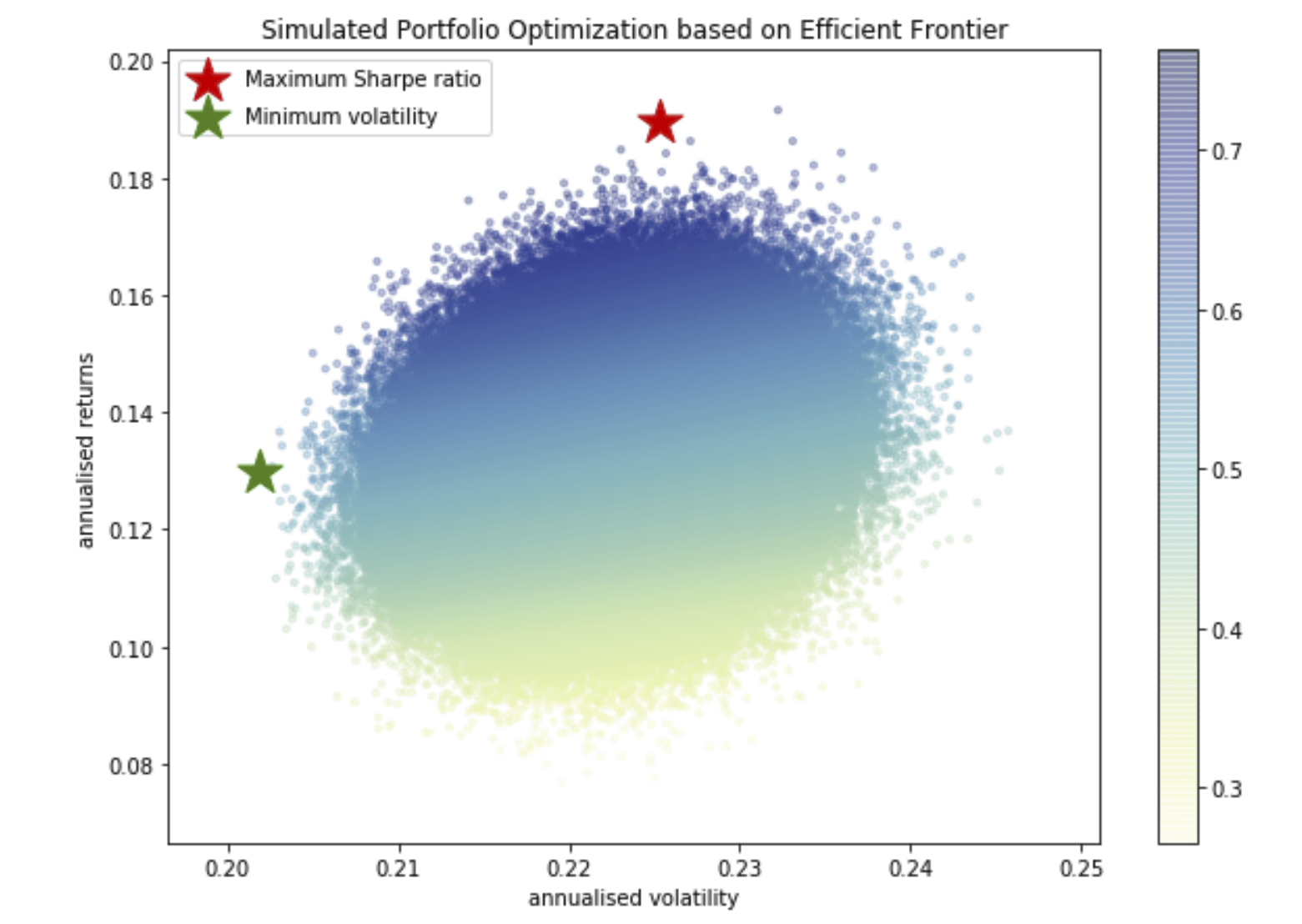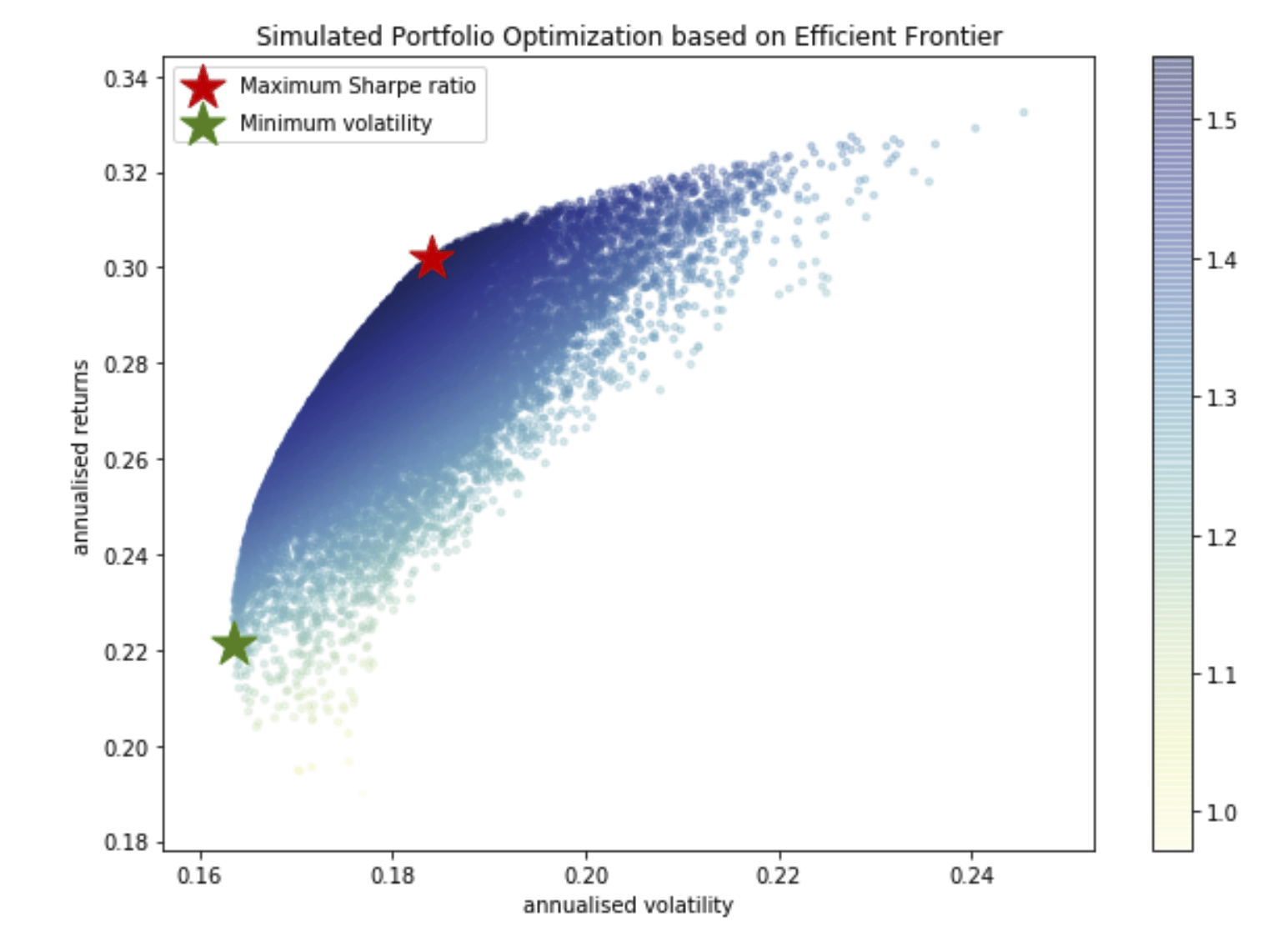# #StackBounty: #python #optimization #heuristic Which heuristic for the simulated portfolio weights?

### Bounty: 50

I am trying to create a Simulated Portfolio Optimization based on Efficient Frontier on 50 stocks, which you can find the csv here. Yet it already takes me several minutes to get a suboptimal solution: I can’t draw an accurate efficient frontier:Whereas it should be something like:So basically, I want to create an efficient frontier of optimization of the weights $$w_i$$ of stocks in a portfolio of actions $$i$$ which returns are $$x_i$$.

I have imagined there is another way to get the weights in the following way. It should be easier to get that efficient frontier getting weights with given, fixed, portfolio standard deviations $$sigma_p$$. Indeed, one can fix a grid of volatilities $$σ{p_1},…σ{p_n}$$, then for each $$σ{p_i}$$, maximize expected returns with the constraint that the volatility is no larger than $$σ$${p_i}\$, to get $$μ{p_i}$$. Then $$(σ$${p_i},μ_{p_i})\$ are $$n$$ points on the efficient frontier.

So, a first step would be to get the weights for one volatility $$σ_{p}$$. Knowing that for two assets, the portfolio variance $$sigma_p$$ is

begin{align} sigma_p &= sqrt{w_1^2sigma_1^2 + w_2^2sigma_2^2 +2w_1w_2cov(x_1,x_2)}\ end{align}

Where $$forall ineq p,sigma_i$$ are the standard deviations for a given asset.

We can maximize returns $$r$$ which are equals to the weights time the individual results for each action $$RW$$. This leads to the following optimization problem (I reduced it to two variables for the sake of simplicity):

$$begin{cases}max r\ &sigma_p leq value\ &sigma_p = sqrt{w_1^2sigma_1^2+w_2^2sigma^2+2w_1w_2cov_{1,2}}\ &r = w_1r_1+w_2r_2\ &forall i, w_igeq 0 end{cases}$$

I don’t know how to write it in matrix formulation:

$$begin{cases}max r\ &sigma_p leq value\ &sigma_p = sqrt{W^2Sigma^2+2WW^TCOV}\ &r = WR\ &forall i, w_igeq 0 end{cases}$$

Where COV is the covariance matrix between all assets.

But I don’t know if it’s right and how to write it in python.

# Context

My original approach was naive sampling. It doesn’t work well because the efficient frontier is a very small subspace of the space I’m exploring:

``````import pandas as pd
import numpy as np
import matplotlib.pyplot as plt
import seaborn as sns
import quandl
#import scipy.optimize as scoplt.style.use('fivethirtyeight')
np.random.seed(777)

def portfolio_annualised_performance(weights, mean_returns, cov_matrix):
returns = np.sum(mean_returns*weights ) *252
std = np.sqrt(np.dot(weights.T, np.dot(cov_matrix, weights))) * np.sqrt(252)
return std, returns

def random_portfolios(num_portfolios, mean_returns, cov_matrix, risk_free_rate, df):
results = np.zeros((3,num_portfolios))
weights_record = []
for i in range(num_portfolios):
weights = np.random.random(len(df.columns))
weights /= np.sum(weights)
weights_record.append(weights)
portfolio_std_dev, portfolio_return = portfolio_annualised_performance(weights, mean_returns, cov_matrix)
results[0,i] = portfolio_std_dev
results[1,i] = portfolio_return
results[2,i] = (portfolio_return - risk_free_rate) / portfolio_std_dev
return results, weights_record

def display_simulated_ef_with_random(mean_returns, cov_matrix, num_portfolios, risk_free_rate, df):
results, weights = random_portfolios(num_portfolios,mean_returns, cov_matrix, risk_free_rate, df)

max_sharpe_idx = np.argmax(results)
sdp, rp = results[0,max_sharpe_idx], results[1,max_sharpe_idx]
print("results[0,max_sharpe_idx], results[1,max_sharpe_idx]: ", results[0,max_sharpe_idx], results[1,max_sharpe_idx])
max_sharpe_allocation = pd.DataFrame(weights[max_sharpe_idx],index=df.columns,columns=['allocation'])
max_sharpe_allocation.allocation = [round(i*100,2)for i in max_sharpe_allocation.allocation]
max_sharpe_allocation = max_sharpe_allocation.T

min_vol_idx = np.argmin(results)
sdp_min, rp_min = results[0,min_vol_idx], results[1,min_vol_idx]
min_vol_allocation = pd.DataFrame(weights[min_vol_idx],index=df.columns,columns=['allocation'])
min_vol_allocation.allocation = [round(i*100,2)for i in min_vol_allocation.allocation]
min_vol_allocation = min_vol_allocation.T

print("-"*80)
print("Maximum Sharpe Ratio Portfolio Allocationn")
print("Annualised Return:", round(rp,2))
print("Annualised Volatility:", round(sdp,2))
print("n")
print(max_sharpe_allocation)
print("-"*80)
print("Minimum Volatility Portfolio Allocationn")
print("Annualised Return:", round(rp_min,2))
print("Annualised Volatility:", round(sdp_min,2))
print("n")
print(min_vol_allocation)

plt.figure(figsize=(10, 7))
plt.scatter(results[0,:],results[1,:],c=results[2,:],cmap='YlGnBu', marker='o', s=10, alpha=0.3)
plt.colorbar()
plt.scatter(sdp,rp,marker='*',color='r',s=500, label='Maximum Sharpe ratio')
plt.scatter(sdp_min,rp_min,marker='*',color='g',s=500, label='Minimum volatility')
plt.title('Simulated Portfolio Optimization based on Efficient Frontier')
plt.xlabel('annualised volatility')
plt.ylabel('annualised returns')
plt.legend(labelspacing=0.8)

return max_sharpe_allocation, min_vol_allocation

returns = df.pct_change()
mean_returns = returns.mean()
cov_matrix = returns.cov()
num_portfolios = 750000
risk_free_rate = 0.0178

min_vol_al, max_sharpe_al = display_simulated_ef_with_random(mean_returns, cov_matrix, num_portfolios, risk_free_rate, df)
``````

As a side note, one should also notice that:

``````std = np.sqrt(np.dot(weights.T, np.dot(cov_matrix, weights))) * np.sqrt(252)
``````

Which leads to another equation which I don’t know if it can be useful:

$$W^TCW = (frac{sigma_p}{sqrt{252}})^2$$

# solving a linear system

The minimizer of a quadratic objective under linear constraints can be obtained by solving a linear system. Which is really easy to compute and not that hard to understand. To get the best sharpe ratio it gives the following:

``````def efficient_portfolios(returns, risk_free_rate, sigma, mu, e):
weights_record = []
volatilities = []
results = np.zeros((3,len(returns)))
i = 0
for portfolio_return in returns:
A = np.block([[2*sigma, mu, e], [mu.T, 0, 0], [e.T, 0, 0]])
b = np.zeros(n+2)
b[n] = portfolio_return
b[n+1] = 1
w = np.linalg.solve(A, b)[:n]
weights_record.append(w)
portfolio_std_dev = np.sqrt( w.T @ sigma @ w )
volatilities.append(portfolio_std_dev)
results[0,i] = portfolio_std_dev
results[1,i] = portfolio_return
results[2,i] = (portfolio_return - risk_free_rate) / portfolio_std_dev
i+=1
return results, weights_record, volatilities

def display_simulated_ef_with_random(mean_returns, risk_free_rate, sigma, mu, e, df):
results, weights, volatilities = efficient_portfolios(mean_returns,risk_free_rate, sigma, mu, e)
max_sharpe_idx = np.argmax(results)
sdp, rp = results[0,max_sharpe_idx], results[1,max_sharpe_idx]
max_sharpe_allocation = pd.DataFrame(weights[max_sharpe_idx],index=df.columns,columns=['allocation'])
max_sharpe_allocation.allocation = [round(i*100,2)for i in max_sharpe_allocation.allocation]
max_sharpe_allocation = max_sharpe_allocation.T

min_vol_idx = np.argmin(results)
sdp_min, rp_min = results[0,min_vol_idx], results[1,min_vol_idx]
min_vol_allocation = pd.DataFrame(weights[min_vol_idx],index=df.columns,columns=['allocation'])
min_vol_allocation.allocation = [round(i*100,2)for i in min_vol_allocation.allocation]
min_vol_allocation = min_vol_allocation.T

print("-"*80)
print("Maximum Sharpe Ratio Portfolio Allocationn")
print("Annualised Return:", round(rp,2))
print("Annualised Volatility:", round(sdp,2))
print("n")
print(max_sharpe_allocation)
print("-"*80)
print("Minimum Volatility Portfolio Allocationn")
print("Annualised Return:", round(rp_min,2))
print("Annualised Volatility:", round(sdp_min,2))
print("n")
print(min_vol_allocation)

plt.figure(figsize=(10, 7))
plt.scatter(results[0,:],results[1,:],c=results[2,:],cmap='YlGnBu', marker='o', s=10, alpha=0.3)
plt.colorbar()
plt.scatter(sdp,rp,marker='*',color='r',s=500, label='Maximum Sharpe ratio')
plt.scatter(sdp_min,rp_min,marker='*',color='g',s=500, label='Minimum volatility')
plt.title('Simulated Portfolio Optimization based on Efficient Frontier')
plt.xlabel('annualised volatility')
plt.ylabel('annualised returns')
plt.legend(labelspacing=0.8)

return max_sharpe_allocation, min_vol_allocation
``````

And it gives the following plot and portfolios:

``````--------------------------------------------------------------------------------
Maximum Sharpe Ratio Portfolio Allocation

Annualised Return: 0.63
Annualised Volatility: 0.23

allocation -19.33  0.03 -0.32  29.3  12.65 -14.57  2.85 -25.28 -13.17  2.77

...   SWKS    NOV  KMT   MDT   RIO   PSA   STE  POWI  VALE   TX
allocation  ... -15.61 -10.08 -7.2 -3.16  7.57 -9.39  7.93  5.13  1.07  8.4

[1 rows x 51 columns]
--------------------------------------------------------------------------------
Minimum Volatility Portfolio Allocation

Annualised Return: 0.03
Annualised Volatility: 0.13

allocation -0.6 -7.11  5.36  3.81  22.9 -3.69  7.37 -1.27 -1.13 -0.16  ...

SWKS   NOV   KMT   MDT   RIO    PSA   STE  POWI  VALE    TX
allocation  -6.4 -0.25 -9.24  6.15  4.41  19.86 -1.31 -0.23 -2.99  6.05
``````Let me know if I did anything wrong.

But how would you do if we needed w to always be positive? I know that the solution cannot simply be obtained by solving a linear system. I would need to use a quadratic optimization solver.

Get this bounty!!!

This site uses Akismet to reduce spam. Learn how your comment data is processed.